# Factors multiple primes Prime factors Truefalse Reflect Your

• Slides: 5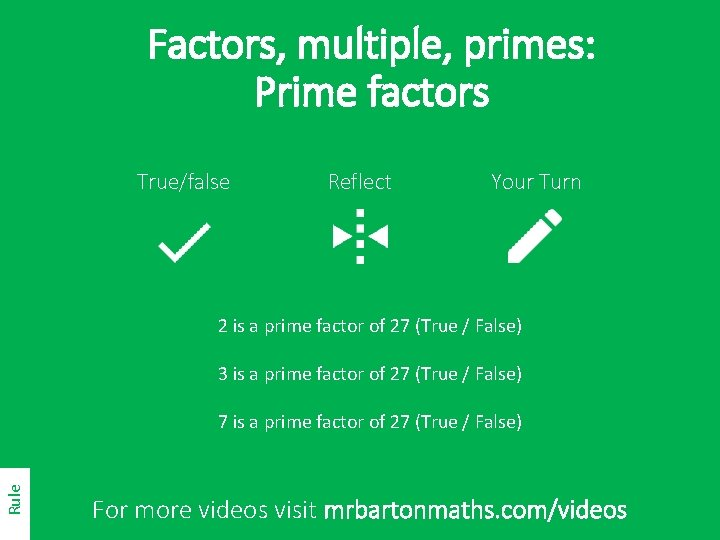Factors, multiple, primes: Prime factors True/false Reflect Your Turn 2 is a prime factor of 27 (True / False) 3 is a prime factor of 27 (True / False) Rule 7 is a prime factor of 27 (True / False) For more videos visit mrbartonmaths. com/videos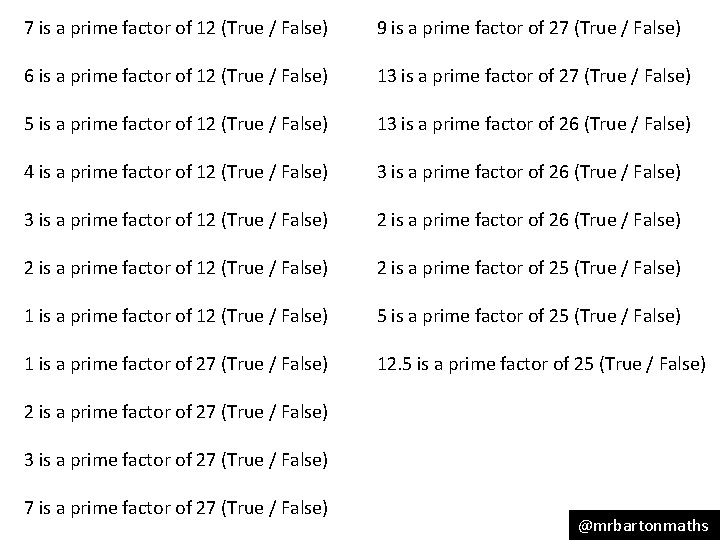7 is a prime factor of 12 (True / False) 9 is a prime factor of 27 (True / False) 6 is a prime factor of 12 (True / False) 13 is a prime factor of 27 (True / False) 5 is a prime factor of 12 (True / False) 13 is a prime factor of 26 (True / False) 4 is a prime factor of 12 (True / False) 3 is a prime factor of 26 (True / False) 3 is a prime factor of 12 (True / False) 2 is a prime factor of 26 (True / False) 2 is a prime factor of 12 (True / False) 2 is a prime factor of 25 (True / False) 1 is a prime factor of 12 (True / False) 5 is a prime factor of 25 (True / False) 1 is a prime factor of 27 (True / False) 12. 5 is a prime factor of 25 (True / False) 2 is a prime factor of 27 (True / False) 3 is a prime factor of 27 (True / False) 7 is a prime factor of 27 (True / False) @mrbartonmaths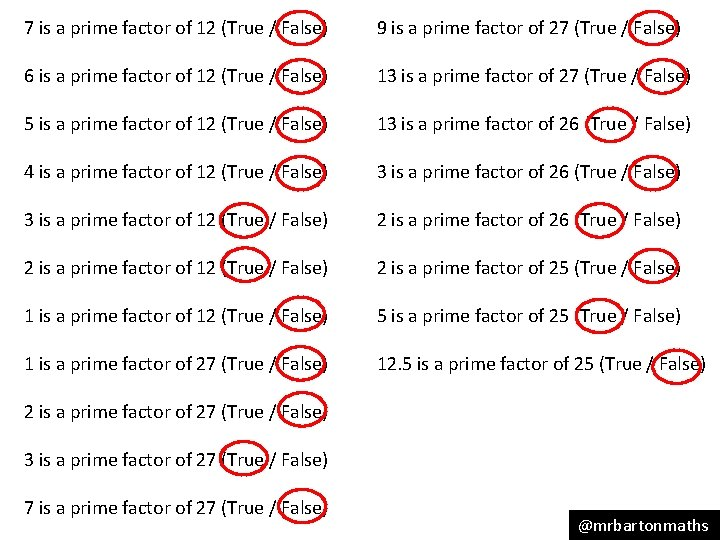7 is a prime factor of 12 (True / False) 9 is a prime factor of 27 (True / False) 6 is a prime factor of 12 (True / False) 13 is a prime factor of 27 (True / False) 5 is a prime factor of 12 (True / False) 13 is a prime factor of 26 (True / False) 4 is a prime factor of 12 (True / False) 3 is a prime factor of 26 (True / False) 3 is a prime factor of 12 (True / False) 2 is a prime factor of 26 (True / False) 2 is a prime factor of 12 (True / False) 2 is a prime factor of 25 (True / False) 1 is a prime factor of 12 (True / False) 5 is a prime factor of 25 (True / False) 1 is a prime factor of 27 (True / False) 12. 5 is a prime factor of 25 (True / False) 2 is a prime factor of 27 (True / False) 3 is a prime factor of 27 (True / False) 7 is a prime factor of 27 (True / False) @mrbartonmaths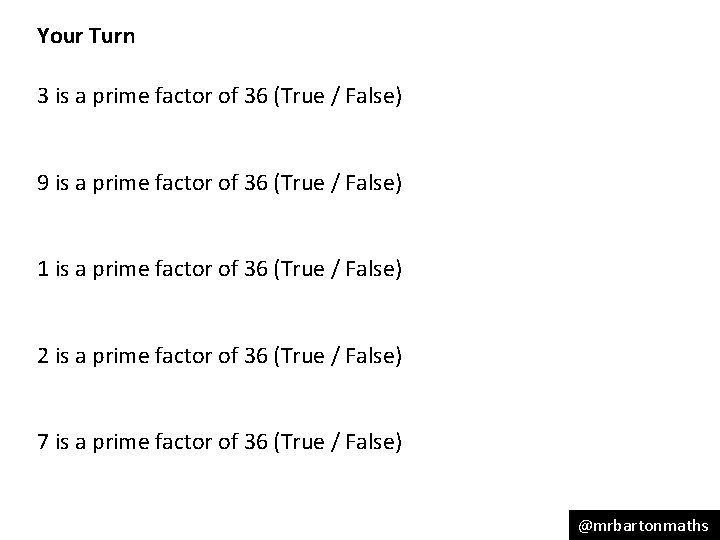Your Turn 3 is a prime factor of 36 (True / False) 9 is a prime factor of 36 (True / False) 1 is a prime factor of 36 (True / False) 2 is a prime factor of 36 (True / False) 7 is a prime factor of 36 (True / False) @mrbartonmaths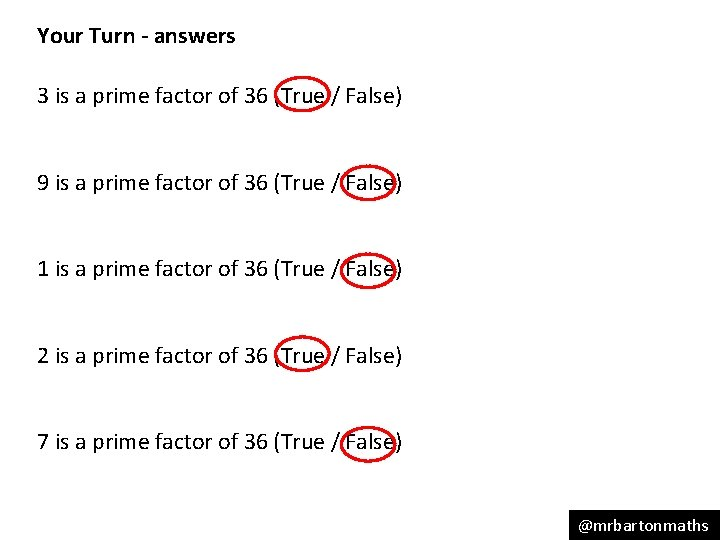Your Turn - answers 3 is a prime factor of 36 (True / False) 9 is a prime factor of 36 (True / False) 1 is a prime factor of 36 (True / False) 2 is a prime factor of 36 (True / False) 7 is a prime factor of 36 (True / False) @mrbartonmaths